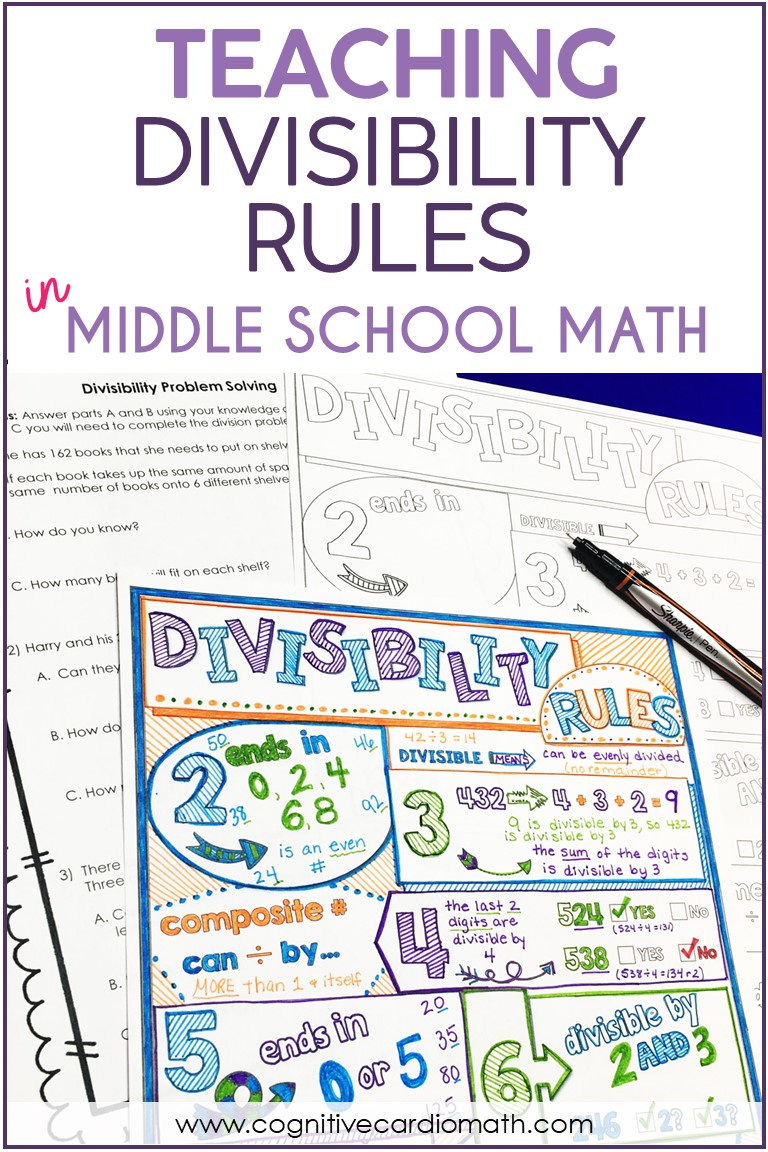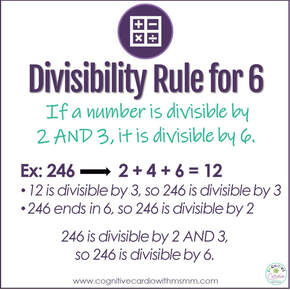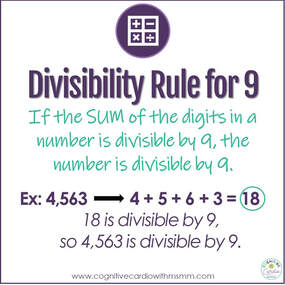# Teaching Divisibility Rules in Middle School Math

## Teaching Divisibility Rules

Do you teach divisibility rules in your middle school math classes?

I’ve always enjoyed teaching divisibility rules, and my 6th grade math students have always seemed to have fun using them!

I’ve read different opinions about whether or not teaching divisibility rules should be a focus in math class, because they may be viewed as ‘tricks.’ I do not view them as tricks, but as a way to develop relationships between numbers.I think understanding and using divisibility rules in middle school helps students develop number sense and number fluency.

Rather than being taught as a unit, I think divisibility rules should be introduced and then referred to again and again in any applicable situation throughout the year.

To make the continuous revisiting easier for students, I’ve always liked to have a resource for them to refer to throughout the year. We used to create fold-it-ups, but then I moved to using a Doodle Notes resource or a Math Wheel.

Any reference sheet is helpful so that when you ask a divisibility question, students can grab it (or look at it on a wall) to quickly refresh their memories, if needed.

## Where Can Students Apply Divisibility Rules?

There are several math concepts where applying divisibility rules will help students:

1) Working with division facts that are beyond the ‘basics’

• Example: 51 divided by 3
• Students so often believe 51 is prime, but if they take a second to test the rule for 3, they can quickly see that 51 is in fact a composite number.

2) Prime factorization

• When determining the prime factorization of a number like 51, 57 or 87, using the divisibility rules can be very helpful.

3) GCF

• To find GCF, students need to determine what each number can be divided by, so the divisibility rules are quite helpful here.

4) FACTORING

• If you teach divisibility rules in elementary school, you might not be thinking of this eventual application. However, the more frequently math students work with divisibility rules, the more their number fluency improves, and the easier factoring will be for them.

## Methods for Teaching Divisibility

As I mentioned above, I think divisibility rules are learned and retained most effectively when they are introduced and then referred to on a regular basis.

It’s also helpful when students have a resource to refer to if they need a quick reminder, so my favorite way to teach the rules are to use the Doodle Notes or the Math WheelThese methods are similar because they both incorporate coloring, doodling, and visual memory triggers. I also love these because they:

1. Increase focus and retention during instruction, by incorporating coloring and doodling (this activates both hemispheres of the brain).
2. Are those year-long resources students can keep in their interactive notebooks.

## What Are the Rules?

In case you’re teaching divisibility rules for the first time (or haven’t taught them in a long time), I’ve included the divisibility rules for 2, 3, 4, 5, 6, 9, and 10 in this post.

There are rules for 7 and 8 as well, but I don’t normally teach these, as they’re a bit more complicated and maybe a little less useful.

2: if a number ends in a 0, 2, 4, 6, 8, it is divisible by 2

• Example: the number 358 ends in 8, so 358 is divisible by 2

3: if the sum of the digits in a number is divisible by 3, the number is divisible by 3

• Example: the sum of the digits in the number 4,563 is 18. 18 is divisible by 3, so 4,563 is divisible by 3.4: if the last 2 digits of a number are divisible by 4, the number is divisible by 4

• Example: in the number 248, the last 2 digits (48) are divisible by 4, so 248 is divisible by 4.

5: if a number ends in 0 or 5, it is divisible by 5

• Example: the number 255 ends in 5, so 255 is divisible by 56: if a number is divisible by 2 AND 3, it is divisible by 6

• ​Example: the sum of the digits in 246 is 12. 12 is divisible by 3, so 246 is divisible by 3
• 246 ends in 6, so 246 is divisible by 2
• 246 is divisible by 2 AND 3, so 246 is divisible by 6.9: if the sum of the digits in a number is divisible by 9, the number is divisible by 9

• Example: the sum of the digits in the number 4,563 is 18. 18 is divisible by 9, so 4,563 is divisible by 9.

10: if a number ends in 0, it is divisible by 10

• Example: the number 4,040 ends in 0, so 4,040 divisible by 10Note:
Finding the sum for the divisibility rules for 3 and 9 sometimes leads some students to start adding the digits to check for every
number.
I’ve found that the use of visuals with the Doodle Notes or the Math Wheel reduced this tendency, as compared to when we used the fold-it ups.

## Ellie

•I like this as a review for my 7th & 8th graders. I know they should already know these, but they don’t. This is a fun way to reteach.

•Hi Ellie,

You are right on!!! I am a big advocate for including divisibility rules in middle school, particularly for number sense and number fluency! I have even found this helpful for students who are still struggling to learn basic multiplication facts. And so true! This lays such an awesome foundation for other math concepts you mentioned.

I am also a big advocate for fraction review! They show up everywhere in everyday events and high school math. When doing A.C.T. review with my students I always tell them, be sure you can get the “easy” problems correct … fractions in word problems, geometry … etc.

Great that you have fun materials to help teachers!

Bonnie StephanWelcome to Cognitive Cardio Math! I’m Ellie, a wife, mom, grandma, and dog ‘mom,’ and I’ve spent just about my whole life in school! With nearly 30 years in education, I’ve taught:

• All subject areas in 4th and 5th grades
• Math, ELA, and science in 6th grade (middle school)

I’ve been creating resources for teachers since 2012 and have worked in the elearning industry for about five years as well!